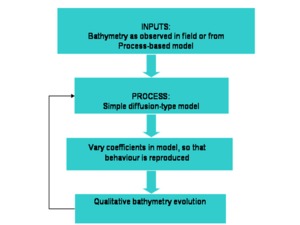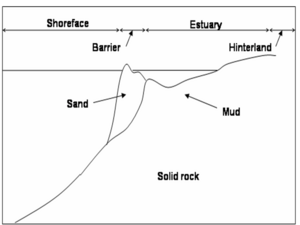# Behaviour-based modelsFigure 1: Schematic showing the steps involved in behaviour-based modelling for long-term studies of bathymetric evolution.

## Contents

### General description

A Behaviour-based model may be defined as a phenomenological model where some of the processes involved in the evolution may have been simplified using semi-empirical averaged formulae (de Vriend 1993, Niedoroda et al. 1995). There is no clear boundary between process-based morphological models and behaviour-based models, except for the fact that in process-based models the hydrodynamics and transport processes are clearly integrated in the model (to the best knowledge or for the purposes of the modeller), and that the parameters are assumed to be constant or some simple function of space and time (in most cases they are assumed as constant, for simplicity). However, when many details are involved, the computations are very difficult to achieve. Also, process-based models are good at describing the dynamics at short time scales, but mechanisms relevant at short time scales may not dominate at longer scales, and in such cases behaviour-based models can be advantageous both from a practical and a scientific perspective. A behaviour-based model formulation is schematised in Figure 1, showing the different steps involved when modelling using a simplified approach. A similar schematic could describe a process-based model, except for the filter of the boundary conditions and the parameter estimations. Indeed, the model may initially use bathymetry inputs from observations, or an explicit form of the bathymetry as predicted from a process-based model. The bathymetry is then updated, and compared to available data. If the prediction is not accurate enough, the model parameters are adjusted. Also, assumptions are made on the most important physical processes and how affect the evolution the system under study. For instance, in 1-line and N-line models in general (see Littoral drift and shoreline modelling ), it is assumed that the shoreline or underwater lines are in equilibrium, and that diffusion is the dominant process for cross-shore transport. In such a model the diffusion coefficient is adjusted until the model reproduces the behaviour to a required accuracy. Many behaviour-based models are numerical in nature, due to the complexity of measured boundary conditions. A few examples are given in the next section.Figure 2: Schematic of some of morphological units that could be included in a shoreface model, such as those by Cowell et al (1995)  and Stolper et al. (2005). Based on Stolper et al. (2005).

### Examples

Behaviour-based models have been widely used in long-term coastal morphodynamics, due to the difficulty in assessing which processes are of relevance in decadal time scales. Most of these model use simplifications or heuristic estimates of some sort, be it in the parameter estimation or in the initial boundary conditions, and rely heavily on numerical computations. The Shoreface Translation Model (STM) developed by Cowell et al. (1992, 1995) is one of such models, where predictions are made using parameter estimates and initial bathymetric profiles based on data. The model assesses time and space evolution of the beach and bathymetric profile based on sediment budgets, sediment substrate composition, and sea level rise. Another behaviour-based model has been developed by Stolper et al. (2005) to analyse morphological long-term evolution due to sea level rise and sediment exchanges between the shoreface, a barrier, and an estuary. The conservation equations of Stolper et al. (2005) and Cowell et al. (1995) are of the same form, but Stolper et al. (2005) consider different stratigraphic components characterised not only by their substrate composition, but also by their erodibility. A schematic of some of the morphological components shoreface models may include is shown in Figure 2. Also, while Cowell et al. (1995) are interested in shoreface evolution for times in the order of decades, Stolper et al. (2005) study shoreface evolution occurring in millennia. This shows the vast time range for which a behaviour-based model may be applicable, in contrast to process-based models which usually cannot deal with such long timescales.

### Related articles

How to apply models
Coastal tract modelling
Process-based morphological models